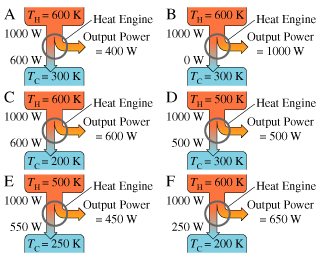# Problem: As part of your job at the patent office, you are asked to evaluate the six designs shown in the figure for innovative new heat engines. (Figure 1)Part AWhich of the designs violate(s) the first law of thermodynamics?Give the letter(s) of the design(s) in alphabetical order, without commas or spaces (e.g., ACD).

###### FREE Expert Solution

The first law of Thermodynamics is expressed as:

$\overline{){\mathbf{∆}}{\mathbf{U}}{\mathbf{=}}{\mathbf{Q}}{\mathbf{+}}{\mathbf{W}}}$, where ΔU is the change in internal energy, Q is the heat transfer, and W is the amount of work done.

There must be a conservation of energy.

That is, the heat input must be the same as heat out + work done.

For A, 600 + 400 = 1000 (Obeys)

79% (500 ratings)###### Problem Details

As part of your job at the patent office, you are asked to evaluate the six designs shown in the figure for innovative new heat engines. (Figure 1)

Part A

Which of the designs violate(s) the first law of thermodynamics?

Give the letter(s) of the design(s) in alphabetical order, without commas or spaces (e.g., ACD).